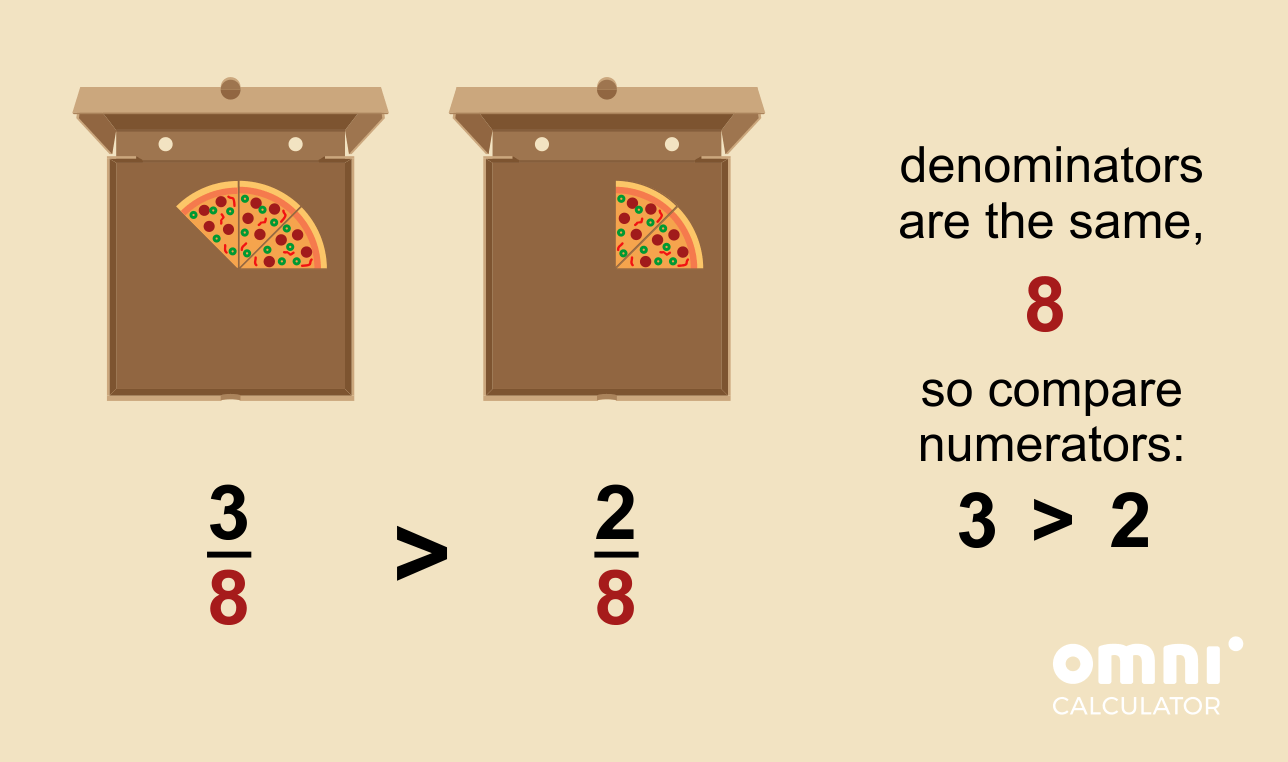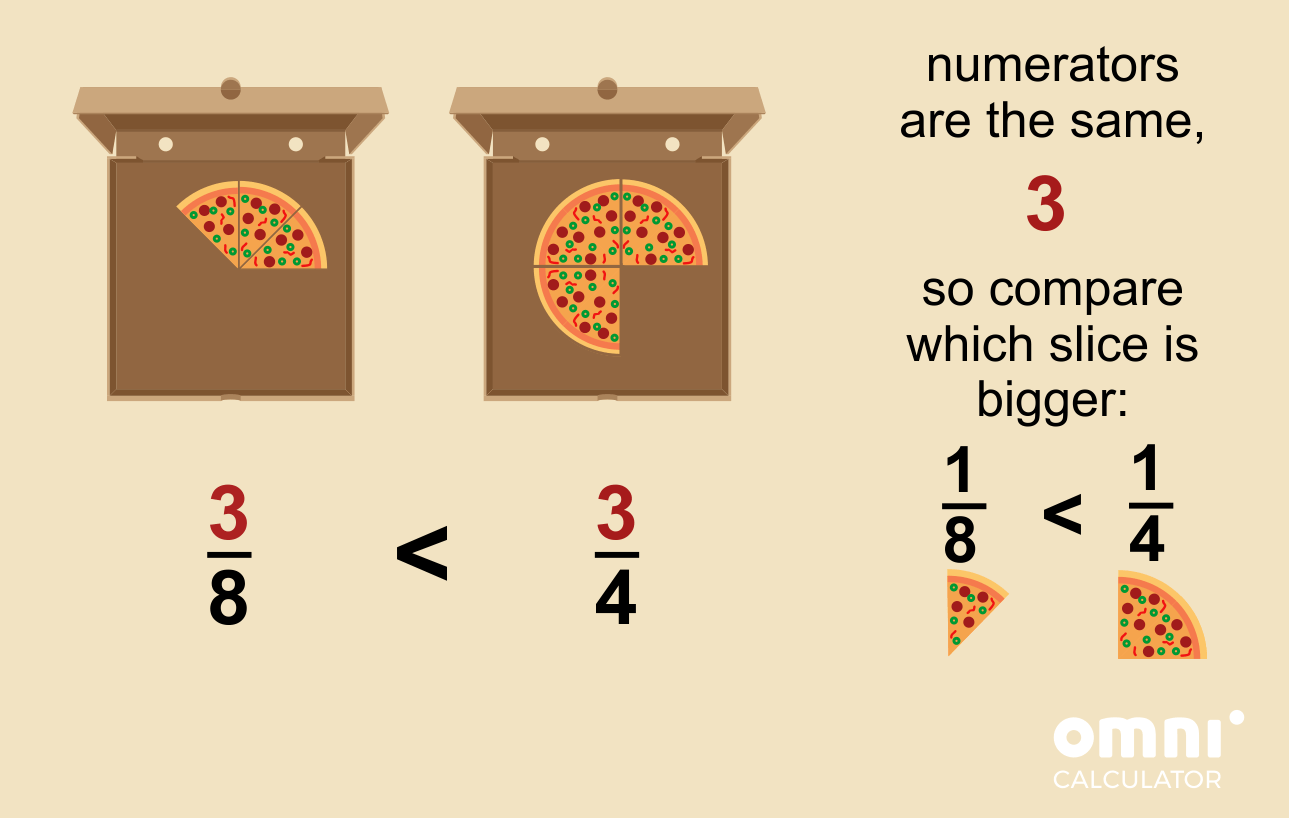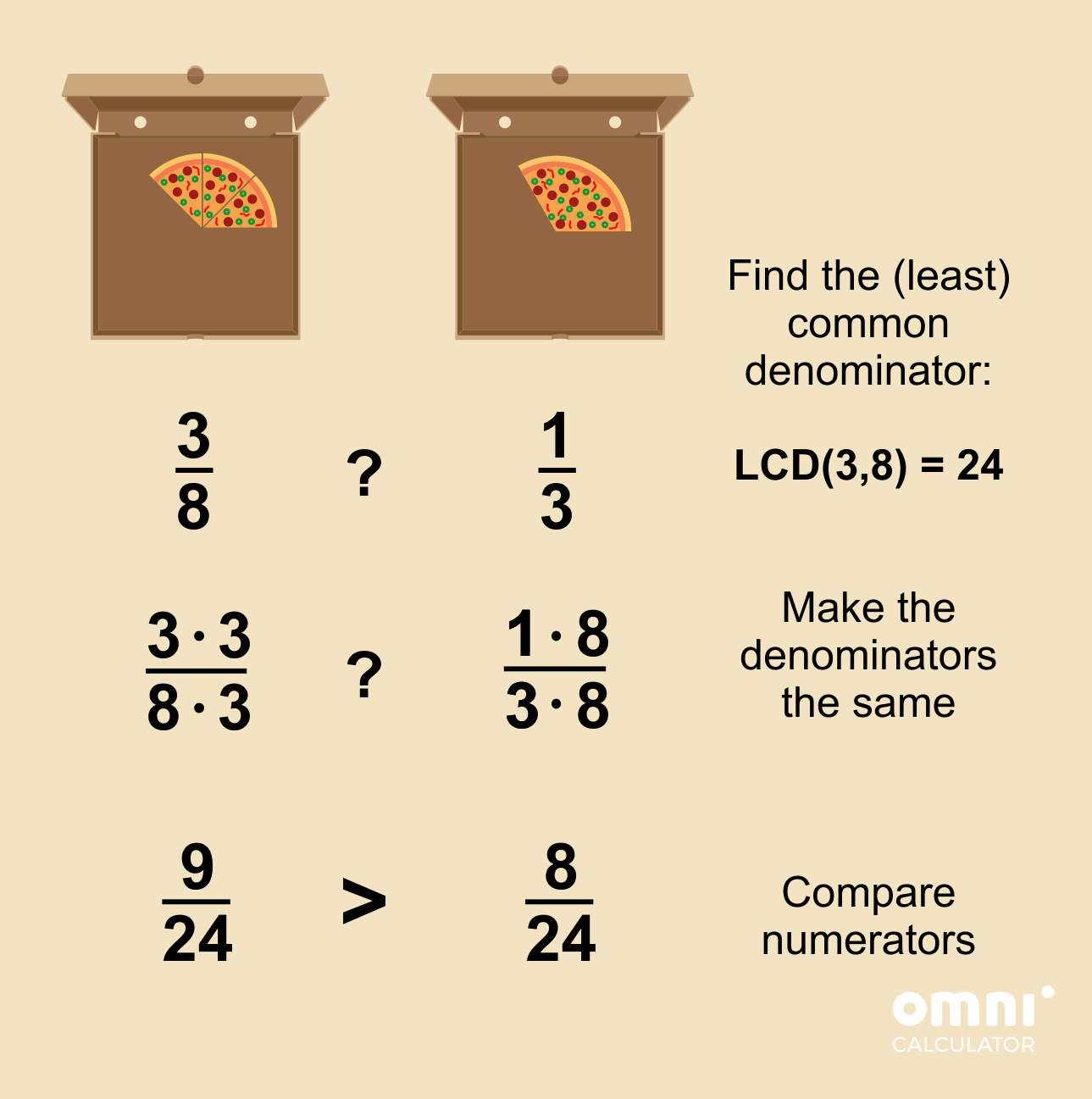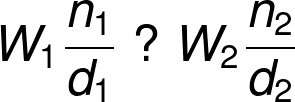# Comparing Fractions Calculator

Created by Hanna Pamuła, PhD candidate
Reviewed by Dominik Czernia, PhD candidate and Jack Bowater
Last updated: Apr 06, 2022

Use this comparing fractions calculator when you are struggling to compare fractions - be they simple fractions, improper fractions, mixed numbers, or even whole numbers. Your fractions don't need to have the same denominator; the tool works perfectly even when comparing fractions with unlike denominators. Like to learn the rules of how to compare fractions? Look no further! 🔍

We'll explain in detail how comparing fractions with the same denominator works, as well as comparing fractions with the same numerator.

## How to compare fractions?

There are two popular methods that you can use to compare fractions:

1. Decimal method - where you convert each fraction to a decimal. It's easy when you have a simple pocket calculator🖩 or our fraction to decimal converter, so we won't go into details here; and
2. Same denominator method - which we describe thoroughly below, as there's a high chance you're searching for this method 😉

So, in the same denominator method, a few cases may occur:

• Comparing fractions with the same denominator - that's the most straightforward case, of course 😀
• Comparing fractions with the same numerator - also, not a tricky problem 🙂
• Comparing fractions with unlike denominators - may be the most problematic, as you need to find the common denominator or least common denominator. However, it's still a piece of cake, trust us! 🍰

In the paragraphs below, we describe in detail how to compare fractions in each of those cases. Scroll down and find a real-life examples - the battle for each and every pizza slice.

## Comparing fractions with the same denominator

Comparing fractions with the same denominator is the easiest option. If two of your fractions have the same denominator (bottom number), the greater fraction will be the one with bigger numerator (top number). So, for example:

4/5 > 3/5, because 4 is greater than 3; and

3/7 < 6/7, because 3 is smaller than 6.

Think of it this way:

We have a lot of slices of pizza🍕, each slice is the same size. Who has more slices, Michael with 3 slices or Emily with 2 slices? Assuming that we cut each pizza to 8 equal slices: ³/₈ > ²/₈.For sure, it's Michael! Three slices 🍕🍕🍕 are definitely more than two slices 🍕🍕, right?

## Comparing fractions with the same numerator

So, what happens if two fractions have the same numerator? Then, the greater fraction is the one with smaller denominator, e.g.

4/5 > 4/7

3/11 < 3/8

Coming back to the pizza analogy:

 Emily didn't like the idea that Michael took more pizza slices. So, she told everyone that, next time, everybody takes the same number of slices - but her foxy plan was that the slices are not equal in size😈. She cut one pizza to 8 slices - and gave Michael 3 slices, and she cut the other pizza to 4 slices and took 3 slices for herself. Who has more pizza? The answer: 3/8 < 3/4Emily took three bigger slices, so she definitely has more pizza than Michael!

## Comparing fractions with unlike denominators

Getting to the heart of the matter - how to compare fractions with unlike denominators, e.g. 3/8 and 1/6 ? There are two methods to make the denominator the same:

1. Common denominator method, where you multiply the top and bottom part of the fraction by the denominator of the other fraction:

3/8 ? 1/6

3/8 ⋅ 6/6 ? 1/6 ⋅ 8/8

18/48 > 8/48

1. Least common denominator method (LCD), where you find the LCM of the denominators and then the equivalent fractions of both fractions with the LCM as the denominator

3/8 ? 1/6

LCM(8,6) = 24

9/24 > 4/24

The methods are pretty similar, and you can use whichever you prefer.

Let's go back to our pizza story:

 Michael get a bit offended by Emily's foul game. Next time they met, he asked Emily directly which one she prefers - having 3 slices out of 8, or 1 slice out of 3? By quick calculations in her head, she has chosen the first option. Was it a good choice?3/8 ? 1/39/24 > 8/24Yes, it was! The difference is not so huge in this case, but the more pizza, the better! 😋

## How to compare fractions - mixed fractions & other cases

In the above paragraphs we described cases where you compare simple fractions.
But what happens if you want to compare e.g. mixed fractions or improper fractions? For sure, you can use our amazing comparing fractions calculator, it can deal with such problems 😎 But do not worry, we'll explain it here too:

1. Comparing with mixed numbers

When comparing two mixed fractions, sometimes you know immediately which mixed number is bigger - if whole part is bigger, then the mixed number will be bigger, too:

2 1/3 > 1 3/4

If the whole number is the same, compare the fraction next to the whole number, as we did in the :

3 2/7 ? 3 1/4

3 8/28 > 3 7/28

1. Comparing with improper fractions

Comparing two improper fractions works precisely the same as if they were simple fractions!

If you want to compare mixed fractions to improper fractions - make the fractions of the same type:

To sum up, the universal solution is:

• Find a common denominator of your fractions. If one of the fractions is a mixed number, convert it to an improper fraction.
• Transform the fractions in such a way that they'll have the same common denominator.
• Compare the top numbers - the fraction with the greater numerator is the greater fraction 🎉

## Example: how to use comparing fractions calculator?

We're pretty sure you're a young, intelligent person, and you found out how the comparing fractions calculator works just by playing around with it. But to make things crystal clear💎, we'll show you how to use our tool step by step.

Let's choose a more complicated case - let's compare fractions with unlike denominators. Additionally, one fraction is a mixed number and the other is an improper fraction: 1 5/6 and 20/11.

1. Choose the fraction form. As one of our fraction is a mixed number, choose that option.
2. Input the first fraction. For our example, the whole number is 1, the numerator equals 5 and the denominator is 6.
3. Enter the second fraction. We'll leave the 'whole number' box empty, put 20 as the numerator and 11 as denominator.
4. Tadaa!✨ The comparing fractions calculator shows the result:

1 5/6 is greater than 20/11!

What is more, it shows the reasoning behind this result:

• It converts the mixed number to improper fraction: 1 5/6 = 11/6; and
• Displays the equivalent fractions with the same denominator: 121/66 > 120/66

Isn't it amazing? 😍

Hanna Pamuła, PhD candidate
Fraction form
Mixed number1st fraction
Whole number (W₁)
Numerator (n₁)
Denominator (d₁)
2nd fraction
Whole number (W₂)
Numerator (n₂)
Denominator (d₂)
Result
People also viewed…

### Car crash force

With this car crash calculator, you can find out how dangerous are car crashes.

### Discount

Discount calculator uses a product's original price and discount percentage to find the final price and the amount you save.

### Ellipse area

This ellipse area calculator will let you quickly calculate the area of an oval.

### Perfect square

Perfect square calculator checks whether your number can be created by multiplying two identical integers.